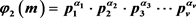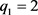﻿ 对一类包含广义欧拉函数的数论方程的讨论 Discussion on a Class of Number Theory Equations Containing Generalized Euler’s Totient Function

Pure Mathematics
Vol. 09  No. 05 ( 2019 ), Article ID: 31403 , 10 pages
10.12677/PM.2019.95081

Discussion on a Class of Number Theory Equations Containing Generalized Euler’s Totient Function

Lu Cai, Changxin Lai, Yanhua Yang

College of Mathematics and Computer Science, Aba Teachers University, Wenchuan Sichuan

Email: 1538249856@qq.com

Received: Jul. 10th, 2019; accepted: Jul. 18th, 2019; published: Jul. 25th, 2019ABSTRACT

Let ${\phi }_{e}\left(n\right)$ be generalized Euler function, where n and e are positive integers. Using elementary methods, the solvability of equations  , ${\phi }_{2}\left(m\right)={p}^{x}\cdot {q}^{y}$ , and ${\phi }_{2}\left(m\right)={p}_{1}^{{\alpha }_{1}}\cdot {p}_{2}^{{\alpha }_{2}}\cdot {p}_{3}^{{\alpha }_{3}}\cdot \cdot \cdot {p}_{v}^{{\alpha }_{v}}$ related to generalized Euler’s totient function is studied and discussed. Based on the properties of generalized Euler’s totient function and Euler’s totient function, the partial solution of the equation is obtained by the discussion of classification and segmentation.

Keywords:Generalized Euler Function, Euler Function, Equation, Positive Integer Solution

Email: 1538249856@qq.com${\phi }_{e}\left(n\right)$ 为广义欧拉函数，其中n与e都是正整数。利用初等方法，研究讨论了与广义欧拉函数有关的数论方程 ${\phi }_{2}\left(m\right)={2}^{x}\cdot {3}^{y}$ 以及的可解性。基于广义欧拉函数的性质，利用初等数论的一些结论以及分类、分段的讨论方式，获得以上方程的正整数解的相关结论。1. 引言与主要结论

Euler函数φ(n)是数论中非常重要而有意义的一类函数，定义为 $1,2,\cdots ,n$ 中与n互素的个数。为了将Lehmer同余式从模素数的平方推广到模任意整数的平方，蔡天新  等对广义Euler函数的作出了如下定义。

${\phi }_{e}\left(n\right)=\underset{\underset{\left(i,1\right)=1}{i=1}}{\overset{\left[\frac{n}{e}\right]}{\sum }}1,$ (1.1)

${\phi }_{\text{2}}\left(n\right)=\frac{1}{2}\phi \left(n\right)$ (1.2)

${\phi }_{\text{2}}\left(m\right)={2}^{x}\cdot {3}^{y}$ (1.3)

${\phi }_{2}\left(m\right)={p}^{x}\cdot {q}^{y}$ (1.4)

${\phi }_{2}\left(m\right)={p}_{\text{1}}^{{\alpha }_{\text{1}}}\cdot {p}_{\text{2}}^{{\alpha }_{\text{2}}}\cdot {p}_{\text{3}}^{{\alpha }_{\text{3}}}\cdot \cdot \cdot {p}_{v}^{{\alpha }_{v}}$ (1.5)Table 1. All nonnegative integer solutions of Equation (1.3) (m, x, y)

1) 若 $p=\text{2}$ 时， $q\ge \text{5}$，有Table 2. In the case of p = 2, q > 5, the nonnegative integer solutions of Equation (1.4) (m, x, y)

2) 若 $p\ne \text{2}$$q\ne \text{3}$，即 $p,q\ge \text{5}$，有Table 3. In the case of p, q ≥ 5, the nonnegative integer solutions of Equation (1.4) (m, x, y)

1) $t=v$，当 ${q}_{t}={p}_{v}$ ( $v=t+\text{1}$ )时， ${\alpha }_{\text{1}}={\beta }_{\text{1}}-\text{2}$${\alpha }_{i}={\beta }_{i}$ ( $i=\left[2,v\right]$ )，且 ${q}_{i}={2}^{{a}_{i}}+1$ ( ${a}_{i}\ge 0$ )。

2) $t>v$，必有 ${q}_{t}>{p}_{v}$${q}_{j}={p}_{j}$$j=\left[1,u\right]$，其中 $u，则 ${\beta }_{u+1},{\beta }_{u+2},\cdots ,{\beta }_{v}=1$，且 ${q}_{i}={2}^{{s}_{{i}_{1}}}\cdot {p}_{2}^{{s}_{{i}_{1}}}\cdot {p}_{3}^{{s}_{{i}_{1}}}\cdot \cdot \cdot {p}_{v}^{{s}_{{i}_{1}}}+1$$i=\left[u+1,v\right]$

2. 方程φ2(m) = 2x3y的解

${\phi }_{\text{2}}\left(m\right)=\frac{1}{2}\phi \left(m\right)$ , ${\phi }_{2}\left(m\right)={2}^{x}\cdot {3}^{y}$

${q}_{1}^{{\beta }_{1}-1}\cdot {q}_{2}^{{\beta }_{2}-1}\cdots {q}_{t}^{{\beta }_{t}-1}\cdot \left({q}_{1}-1\right)\cdot \left({q}_{2}-1\right)\cdots \left({q}_{t}-1\right)={2}^{x+1}\cdot {3}^{y}$ (2.1)

2.1. 情况1：t = 1

$t=1$ 时，有

${q}_{1}^{{\beta }_{1}-1}\cdot \left({q}_{1}-1\right)={2}^{x+1}\cdot {3}^{y}$ (2.2)

2.2. 情况2：t = 2

$t=\text{2}$ 时，有

${q}_{1}^{{\beta }_{1}-1}\cdot \left({q}_{1}-1\right)\cdot {q}_{\text{2}}^{{\beta }_{\text{2}}-1}\cdot \left({q}_{\text{2}}-1\right)={2}^{x+1}\cdot {3}^{y}.$ (2.3)

${q}_{\text{1}}=\text{2}$${q}_{\text{2}}=\text{3}$ 时， ${\text{2}}^{{\beta }_{\text{1}}-\text{1}}\cdot {\text{3}}^{{\beta }_{2}-1}\cdot \text{2}={2}^{x+1}\cdot {3}^{y}$，可得 $m={2}^{a}{3}^{b}$ ( $a\ge 2,b\ge 2$ )， ${q}_{1}={2}^{{s}_{1}}\cdot {3}^{{t}_{1}}+1$ ( ${s}_{1}=0,{t}_{1}=0$ )， ${q}_{2}={2}^{{s}_{2}}\cdot {3}^{{t}_{2}}+1$ ( ${s}_{2}=1,{t}_{2}=0$ )。故 $x={s}_{1}+{s}_{2}+a-2$$y={t}_{1}+{t}_{2}+b-1$

2.3. 情况3：当t ≥ 3时，有

$\underset{i=1}{\overset{t}{\prod }}{q}_{i}^{{\beta }_{i}-1}\cdot \left({q}_{i}-1\right)={2}^{x+1}\cdot {3}^{y}.$ (2.4)

3. 方程 ${\phi }_{2}\left(m\right)={p}^{x}{q}^{y}$ 的解

3.1. 情况A

$p=\text{2}$$q\ge \text{5}$${\phi }_{2}\left(m\right)={2}^{x}{q}^{y}$，则

${q}_{1}^{{\beta }_{1}-1}\cdot {q}_{2}^{{\beta }_{2}-1}\cdots {q}_{t}^{{\beta }_{t}-1}\cdot \left({q}_{1}-1\right)\cdot \left({q}_{2}-1\right)\cdots \left({q}_{t}-1\right)={2}^{x+1}\cdot {q}^{y}.$ (3.1)

$m={q}_{1}^{{\beta }_{1}}\cdot {q}_{2}^{{\beta }_{2}}\cdots {q}_{t}^{{\beta }_{t}}$

${q}_{\text{1}}=\text{2}$${q}_{\text{2}}=q$ 时， ${\text{2}}^{{\beta }_{\text{1}}-\text{1}}\cdot {q}^{{\beta }_{2}-1}\cdot \left(q-1\right)={2}^{x+1}\cdot {q}^{y}$，可得 $m={\text{2}}^{a}{q}^{b}$ ( $a\ge \text{2},b\ge \text{2}$ )， $2={2}^{{s}_{1}}\cdot {q}^{{t}_{1}}+1$ ( ${s}_{\text{1}}=0,{t}_{\text{1}}=0$ )， ${q}_{\text{2}}={2}^{{s}_{\text{2}}}{q}^{{t}_{\text{2}}}+1$ ( ${s}_{2}\ge \text{2},{t}_{2}=0$ )，故 $x={s}_{\text{1}}+{s}_{\text{2}}+a-\text{2}$$y={t}_{\text{1}}+{t}_{\text{2}}+b-\text{1}$

$2={2}^{{s}_{1}}\cdot {q}^{{t}_{1}}+1$ ( ${s}_{\text{1}}=0,{t}_{\text{1}}=0$ )， ${q}_{\text{2}}={2}^{{s}_{\text{2}}}{q}^{{t}_{\text{2}}}+1$${q}_{3}={2}^{{s}_{3}}{q}^{{t}_{\text{3}}}+1$ ( ${s}_{i}\ge \text{1},{t}_{i}\ge 0$ )， $i\ge 2$$t=0$ 时，必有 $s\ge 2$${q}_{\text{2}}\le {q}_{\text{3}}$，故 $x={s}_{1}+{s}_{2}+{s}_{\text{3}}+a-\text{2}$$y={t}_{1}+{t}_{2}+{t}_{\text{3}}$

${q}_{\text{3}}=q$，可得 $m={q}_{\text{1}}{q}_{\text{2}}{q}^{b}$ ( $a\ge \text{2},b\ge \text{2}$ )， ${q}_{1}={2}^{{s}_{1}}{q}^{{t}_{1}}+1$ ( ${s}_{\text{1}}\ge \text{1},{t}_{\text{1}}=0$ )， ${q}_{\text{2}}={2}^{{s}_{\text{2}}}{q}^{{t}_{\text{2}}}+1$ ( ${s}_{2}\ge \text{2},{t}_{2}=0$ )， ${q}_{3}={2}^{{s}_{3}}{q}^{{t}_{\text{3}}}+1$ ( ${s}_{2}\ge \text{2},{t}_{2}=0$ )且 ${q}_{\text{1}}\le {q}_{\text{2}}\le {q}_{\text{3}}$，故 $x={s}_{\text{1}}+{s}_{\text{2}}+{s}_{\text{3}}-\text{1}$$y={t}_{\text{1}}+{t}_{\text{2}}+{t}_{\text{3}}+b-\text{1}$

${q}_{\text{1}}=\text{2}$${q}_{\text{2}}=q$ ( $q\ge \text{5}$，且为质数)时， ${2}^{{\beta }_{1}-1}\cdot {q}^{{\beta }_{2}-1}\cdot \left(q-1\right)\cdot \left({q}_{3}-1\right)={2}^{x+1}\cdot {q}^{y}$，可得， $m={\text{2}}^{a}{q}^{b}{q}_{\text{3}}$ ( $a\ge \text{2},b\ge \text{2}$ )， $2={2}^{{s}_{1}}\cdot {q}^{{t}_{1}}+1$ ( ${s}_{\text{1}}=0,{t}_{\text{1}}=0$ )， ${q}_{\text{2}}={2}^{{s}_{\text{2}}}{q}^{{t}_{\text{2}}}+1$ ( ${s}_{2}\ge \text{1},{t}_{2}=0$ )当 $t=0$ 时，必有 $s\ge 2$${q}_{3}={2}^{{s}_{3}}{q}^{{t}_{\text{3}}}+1$ ( ${s}_{2}\ge 1,{t}_{2}\ge 0$ )当 $t=0$ 时，必有 $s\ge \text{2}$${q}_{\text{2}}\le {q}_{\text{3}}$，故 $x={s}_{1}+{s}_{2}+{s}_{\text{3}}+a-\text{2}$$y={t}_{1}+{t}_{2}+{t}_{\text{3}}+b-\text{1}$

${q}_{j}=q$ ( ${q}_{i}\ge \text{7}$ 且为质数， $i\ge \text{3}$ )时， ${q}^{{\beta }_{\text{1}}-1}\cdot \underset{i=1}{\overset{t}{\prod }}\left({q}_{i}-1\right)={2}^{x+1}\cdot {q}^{y}$，可得 $m={q}^{b}\underset{i=2}{\overset{t}{\prod }}{q}_{i}$${q}_{i}={2}^{{s}_{i}}{3}^{{t}_{i}}+1$ ( ${s}_{i}\ge \text{1},{t}_{i}\ge 0$ )，当 $t=0$ 时， $s\ge 2$，故 $x=\underset{i=\text{2}}{\overset{t}{\sum }}{s}_{i}-1$$y=\underset{i=2}{\overset{t}{\sum }}{t}_{i}+b-1$

3.2. 情况B

$p\ne 2$$q\ne 3$ 时， $p,q\ge \text{5}$${\phi }_{2}\left(m\right)={p}^{x}{q}^{y}$，则

${q}_{1}^{{\beta }_{1}-1}\cdot {q}_{2}^{{\beta }_{2}-1}\cdots {q}_{t}^{{\beta }_{t}-1}\cdot \left({q}_{1}-1\right)\cdot \left({q}_{2}-1\right)\cdots \left({q}_{t}-1\right)=2\cdot {p}^{x}\cdot {q}^{y}$ (3.2)

$p=2\cdot {p}^{{s}_{1}}\cdot {q}^{{t}_{1}}+1$ ( ${s}_{\text{1}}=0,{t}_{\text{1}}=0$ )，故 $x={s}_{\text{1}}$$y={t}_{1}$

4. 方程 ${\phi }_{2}\left(m\right)={p}_{1}^{{\alpha }_{1}}\cdot {p}_{2}^{{\alpha }_{2}}\cdot {p}_{3}^{{\alpha }_{3}}\cdot \cdot \cdot {p}_{v}^{{\alpha }_{v}}$ 的解

${q}_{1}^{{\beta }_{1}-1}\cdot {q}_{2}^{{\beta }_{2}-1}\cdot \cdot \cdot {q}_{t}^{{\beta }_{t}-1}\cdot \left({q}_{1}-1\right)\cdot \left({q}_{2}-1\right)\cdot \cdot \cdot \left({q}_{t}-1\right)={2}^{{\alpha }_{1}+1}\cdot {p}_{\text{2}}^{{\alpha }_{\text{2}}}\cdot {p}_{\text{3}}^{{\alpha }_{\text{3}}}\cdot \cdot \cdot {p}_{v}^{{\alpha }_{v}}$ (4.1)

4.1. 情况1

$t=\text{1}$ 时， ${q}_{1}^{{\beta }_{\text{1}}-1}\cdot \left({q}_{1}-1\right)={2}^{{\alpha }_{1}+1}\cdot {p}_{\text{2}}^{{\alpha }_{\text{2}}}\cdot {p}_{\text{3}}^{{\alpha }_{\text{3}}}\cdot \cdot \cdot {p}_{v}^{{\alpha }_{v}}$ (令 $p\le q$ )

4.2. 情况2

$t=\text{2}$ 时， ${q}_{1}^{{\beta }_{1}-1}\cdot \left({q}_{1}-1\right)\cdot {q}_{2}^{{\beta }_{2}-1}\cdot \left({q}_{1}-1\right)={2}^{{\alpha }_{1}+1}\cdot {p}_{\text{2}}^{{\alpha }_{\text{2}}}\cdot {p}_{\text{3}}^{{\alpha }_{\text{3}}}\cdot \cdot \cdot {p}_{v}^{{\alpha }_{v}}$ (令 $p\le q$ )

4.3. 情况3

$t\ge \text{3}$ 时， $\underset{i=1}{\overset{t}{\prod }}{q}_{i}{}^{{\beta }_{i}-1}\cdot \left({q}_{i}-1\right)={2}^{{\alpha }_{1}+1}\cdot {p}_{\text{2}}^{{\alpha }_{\text{2}}}\cdot {p}_{\text{3}}^{{\alpha }_{\text{3}}}\cdot \cdot \cdot {p}_{v}^{{\alpha }_{v}}$

${\alpha }_{u\text{3}}={s}_{{u}_{\text{3}}}+{b}_{\text{3}}-1$ ( ${s}_{{u}_{1}}=0$ ) ${s}_{{u}_{\text{2}}}$ 之后也为0。

Discussion on a Class of Number Theory Equations Containing Generalized Euler’s Totient Function[J]. 理论数学, 2019, 09(05): 611-620. https://doi.org/10.12677/PM.2019.95081

1. 1. Cai, T.X., Fu, X.D. and Zhou, X. (2007) A Congruence Involving the Quotients of Euler and Its Applications (II). Acta Arithmetica, 130, 203-214.
https://doi.org/10.4064/aa130-3-1

2. 2. Cai, T.X., Shen, Z.Y. and Hu, M.J. (2013) On the Parity of the General-ized Euler Function. Advances in Mathematics, 42, 505-510.

3. 3. Shen, Z.Y., Cai, T.X. and Hu, M.J. (2016) On the Parity of the Generalized Euler Function (II). Advances in Mathematics, 45, 509-519.

4. 4. 俞洪玲, 沈忠燕. 与广义欧拉函数有关的方程[J]. 浙江外国语学院学报, 2012(3): 91-97.

5. 5. 金明艳, 沈忠燕. 方程φ2(n) = 2Ω(n)和φ2(φ2(n)) = 2Ω(n)的可解性[J]. 浙江外国语学院学报, 2013(4): 47-52.

6. 6. 张四保, 陈佳宏, 杨燕妮. 一个包含广义Euler函数φ3(n)方程的解[J]. 科技通报, 2019, 35(3): 7-10.

7. 7. 张四保. 与广义Euler函数有关的一方程的解[J]. 科技通报, 2018, 34(8): 1-5+9.

8. 8. 张四保. 广义Euler函数方程φ6(n) = 2ω(n)的解[J]. 西南师范大学学报(自然科学版), 2018, 43(2): 36-41.

9. 9. 张四保. 数论函数方程φ(φ(n)) = 2ω(n)3ω(n)的奇数解[J]. 西南师范大学学报(自然科学版), 2017, 42(2): 1-4.

10. 10. 张四保. 包含Euler函数φ(n)与函数Ω(n)方程的解[J]. 山西大学学报(自然科学版), 2017, 40(2): 225-228.

11. 11. 多布杰. 关于数论函数方程φ(φ(n)) = 2ω(n)的可解性问题研究[J]. 西藏大学学报(自然科学版), 2012, 27(1): 102-106.

12. 12. 张四保. 与三个数论函数有关的两个方程的解[J]. 数学的实践与认识, 2018, 48(10): 296-300.

13. 13. 陈斌, 吉宇锋. 关于一类包含Euler函数方程的解[J]. 河南科学, 2009, 27(12): 1500-1501.

14. 14. 陈国慧. 一个包含Euler函数的方程[J]. 纯粹数学与应用数学, 2007(4): 439-445+457.

15. 15. 王蓉, 廖群英. 方程 的可解性[J]. 纯粹数学与应用数学, 2016, 32(5): 481-494.

16. 16. 王容. 一类广义欧拉函数及相关方程[D]: [硕士学位论文]. 成都: 四川师范大学, 2018.

17. 17. 王容, 廖群英. 关于广义欧拉函数φ5(n)[J]. 四川师范大学学报(自然科学版), 2018, 41(4): 445-449.

18. 18. 王容, 罗文力, 廖群英. 方程φ3(n) = d/n的可解性[J]. 成都信息工程大学学报, 2017, 32(1): 95-101.

19. 19. 张四保. Euler函数φ(n)与广义Euler函数φ2(n)混合的两个方程[J]. 北华大学学报(自然科学版), 2019, 20(1): 8-14.

20. 20. 张四保, 官春梅, 杨燕妮. 广义Euler函数φ2(n)与Euler函数φ(n)混合的一方程[J]. 数学的实践与认识, 2018, 48(9): 265-268.

21. 21. 张四保. 数论函数方程φ(xy) = kφ(x)φ(y)的正整数解[J]. 黑龙江大学自然科学学报, 2017, 34(2): 181-185.

22. 22. 杨张媛, 赵西卿, 白继文. 一个包含Euler函数的方程[J]. 贵州师范大学学报(自然科学版), 2018, 36(4): 80-88.

23. 23. 白继文, 赵西卿. 与Euler函数有关的一个方程的正整数解[J]. 延安大学学报(自然科学版), 2017, 36(2): 5-7.

24. 24. 郭瑞, 赵西卿. 一类包含Euler函数方程的正整数解[J]. 江汉大学学报(自然科学版), 2016, 44(5): 411-421.

25. 25. 鲁伟阳, 高丽, 王曦浛. 有关Euler函数φ(n)的方程的可解性问题[J]. 江西科学, 2016, 34(1): 15-16+23.

26. 26. 张四保, 杜先存. 一个包含Euler函数方程的正整数解[J]. 华中师范大学学报(自然科学版), 2015, 49(4): 497-501.

27. 27. 高丽, 张佳凡. Euler函数方程φ(xy) = 11(φ(x)+φ(y))的正整数解[J]. 云南师范大学学报(自然科学版), 2017, 37(5): 13-19.

28. 28. 王曦浛, 高丽, 鲁伟阳. 一个包含Euler函数方程的正整数解[J]. 贵州大学学报(自然科学版), 2016, 33(4): 25-29.